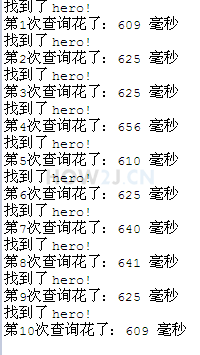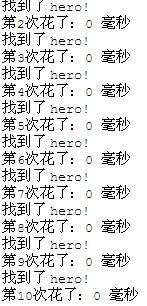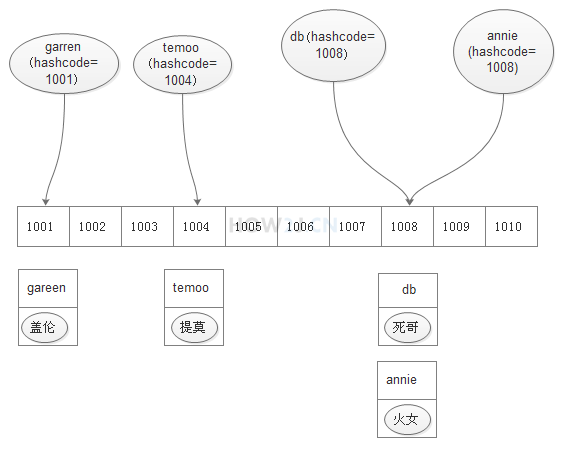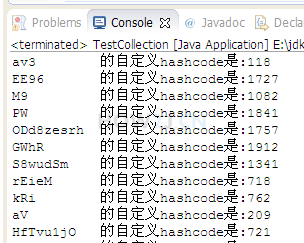集合框架系列教材 （十五）- 关系与区别 - HashSet、 LinkedHashSet、TreeSet之间的区别 集合框架系列教材 （十七）- 其他 - Java Comparator和Comparable 比较器20分40秒

## List查找的低效率

List的做法是对每一个进行挨个遍历，直到找到名字叫做“hero 1000000”的英雄。

1. 初始化2000000个对象到ArrayList中
2. 打乱容器中的数据顺序
3. 进行10次查询，统计每一次消耗的时间package collection; import java.util.ArrayList; import java.util.Collections; import java.util.List; import charactor.Hero; public class TestCollection { public static void main(String[] args) { List<Hero> heros = new ArrayList<Hero>(); for (int j = 0; j < 2000000; j++) { Hero h = new Hero("Hero " + j); heros.add(h); } // 进行10次查找，观察大体的平均值 for (int i = 0; i < 10; i++) { // 打乱heros中元素的顺序 Collections.shuffle(heros); long start = System.currentTimeMillis(); String target = "Hero 1000000"; for (Hero hero : heros) { if (hero.name.equals(target)) { System.out.println("找到了 hero!" ); break; } } long end = System.currentTimeMillis(); long elapsed = end - start; System.out.println("一共花了：" + elapsed + " 毫秒"); } } }

## HashMap的性能表现

1. 初始化2000000个对象到HashMap中。
2. 进行10次查询
3. 统计每一次的查询消耗的时间package collection; import java.util.HashMap; import charactor.Hero; public class TestCollection { public static void main(String[] args) { HashMap<String,Hero> heroMap = new HashMap<String,Hero>(); for (int j = 0; j < 2000000; j++) { Hero h = new Hero("Hero " + j); heroMap.put(h.name, h); } System.out.println("数据准备完成"); for (int i = 0; i < 10; i++) { long start = System.currentTimeMillis(); //查找名字是Hero 1000000的对象 Hero target = heroMap.get("Hero 1000000"); System.out.println("找到了 hero!" + target.name); long end = System.currentTimeMillis(); long elapsed = end - start; System.out.println("一共花了：" + elapsed + " 毫秒"); } } }

## HashMap原理与字典

555相当于就是Lengendary对应的hashcode

## 分析HashMap性能卓越的原因

-----hashcode概念-----

-----保存数据-----

-----查找数据-----## HashSet判断是否重复

HashSet的数据是不能重复的，相同数据不能保存在一起，到底如何判断是否是重复的呢？

hashcode是否一样

## 练习-自定义字符串的hashcode

姿势不对,事倍功半! 点击查看做练习的正确姿势

s*31^(n-1) + s*31^(n-2) + ... + s[n-1]

s 表示第一位字符
n表示字符串的长度

public static int hashcode(String)

(s+ s + s + s+ s[n-1])*23.

## 答案-自定义字符串的hashcode8分57秒 本视频采用html5方式播放，如无法正常播放，请将浏览器升级至最新版本，推荐火狐，chrome，360浏览器。 如果装有迅雷，播放视频呈现直接下载状态，请调整 迅雷系统设置-基本设置-启动-监视全部浏览器 (去掉这个选项)。 chrome 的 视频下载插件会影响播放，如 IDM 等，请关闭或者切换其他浏览器package collection; public class TestCollection { public static void main(String[] args) { for (int i = 0; i < 100; i++) { int length = (int) (Math.random()*8+2); String str = randomString(length); int hashcode = hashcode(str); System.out.printf("%-11s的自定义hashcode是:%d%n",str,hashcode); } } private static int hashcode(String str) { // TODO Auto-generated method stub if(0==str.length()) return 0; int hashcode = 0; char[]cs= str.toCharArray(); for (int i = 0; i < cs.length; i++) { hashcode +=cs[i]; } hashcode*=23; //取绝对值 hashcode = hashcode<0?0-hashcode:hashcode; //落在0-1999之间 hashcode %=2000; return hashcode; } private static String randomString(int length) { String pool = ""; for (short i = '0'; i <= '9'; i++) { pool += (char) i; } for (short i = 'a'; i <= 'z'; i++) { pool += (char) i; } for (short i = 'A'; i <= 'Z'; i++) { pool += (char) i; } char cs[] = new char[length]; for (int i = 0; i < cs.length; i++) { int index = (int) (Math.random() * pool.length()); cs[i] = pool.charAt(index); } String result = new String(cs); return result; } }

## 练习-自定义MyHashMap

姿势不对,事倍功半! 点击查看做练习的正确姿势

MyHashMap内部由一个长度是2000的对象数组实现。

package collection; public interface IHashMap { public void put(String key,Object object); public Object get(String key); }
package collection; //键值对 package collection; //键值对 public class Entry { public Entry(Object key, Object value) { super(); this.key = key; this.value = value; } public Object key; public Object value; @Override public String toString() { return "[key=" + key + ", value=" + value + "]"; } }

## 答案-自定义MyHashMap11分24秒 本视频采用html5方式播放，如无法正常播放，请将浏览器升级至最新版本，推荐火狐，chrome，360浏览器。 如果装有迅雷，播放视频呈现直接下载状态，请调整 迅雷系统设置-基本设置-启动-监视全部浏览器 (去掉这个选项)。 chrome 的 视频下载插件会影响播放，如 IDM 等，请关闭或者切换其他浏览器

## 练习-内容查找性能比较

姿势不对,事倍功半! 点击查看做练习的正确姿势

hero-3229
hero-6232
hero-9365
...

1. 不使用MyHashMap，直接使用for循环找出来，并统计花费的时间
2. 借助MyHashMap，找出结果，并统计花费的时间

## 答案-内容查找性能比较3分3秒 本视频采用html5方式播放，如无法正常播放，请将浏览器升级至最新版本，推荐火狐，chrome，360浏览器。 如果装有迅雷，播放视频呈现直接下载状态，请调整 迅雷系统设置-基本设置-启动-监视全部浏览器 (去掉这个选项)。 chrome 的 视频下载插件会影响播放，如 IDM 等，请关闭或者切换其他浏览器

package collection; import java.util.ArrayList; import java.util.List; import charactor.Hero; public class TestCollection { public static void main(String[] args) { List<Hero> hs =new ArrayList<>(); System.out.println("初始化开始"); for (int i = 0; i < 100000; i++) { Hero h = new Hero( "hero-" + random()); hs.add(h); } //名字作为key //名字相同的hero，放在一个List中，作为value MyHashMap heroMap =new MyHashMap(); for (Hero h : hs) { List<Hero> list= (List<Hero>) heroMap.get( h.name); if(list==null){ list = new ArrayList<>(); heroMap.put(h.name, list); } list.add(h); } System.out.println("初始化结束"); System.out.println("开始查找"); findByIteration(hs); findByMap(heroMap); } private static List<Hero> findByMap(MyHashMap m) { long start =System.currentTimeMillis(); List <Hero>result= (List<Hero>) m.get("hero-5555"); long end =System.currentTimeMillis(); System.out.printf("通过map查找，一共找到%d个英雄，耗时%d 毫秒%n",result.size(),end-start); return result; } private static List<Hero> findByIteration (List<Hero> hs) { long start =System.currentTimeMillis(); List<Hero> result =new ArrayList<>(); for (Hero h : hs) { if(h.name.equals("hero-5555")){ result.add(h); } } long end =System.currentTimeMillis(); System.out.printf("通过for查找，一共找到%d个英雄，耗时%d 毫秒%n", result.size(),end-start); return result; } public static int random(){ return ((int)(Math.random()*9000)+1000); } }

 集合框架系列教材 （十五）- 关系与区别 - HashSet、 LinkedHashSet、TreeSet之间的区别 集合框架系列教材 （十七）- 其他 - Java Comparator和Comparable 比较器

HOW2J公众号，关注后实时获知最新的教程和优惠活动，谢谢。2023-04-07 Integer.parseInt(String.valueOf(c))，char转int报错
 java6663 期望将随机字符转换为int类型来计算hashcode```public static int hashcode(String str) { if (str.length() == 0) return 0; else { char[] cs = str.toCharArray(); int sum = 0; for (char c : cs) { int num = Integer.parseInt(String.valueOf(c)); System.out.println(num); num = num<0 ? 0-num : num; sum += num; } sum *= 23; sum = sum<0 ? 0-sum : sum; sum %= 2000; return sum; } }``` ```Exception in thread "main" java.lang.NumberFormatException: For input string: "H" at java.base/java.lang.NumberFormatException.forInputString(NumberFormatException.java:67) at java.base/java.lang.Integer.parseInt(Integer.java:668) at java.base/java.lang.Integer.parseInt(Integer.java:786) at collection.TestCollection.hashcode(TestCollection.java:24) at collection.TestCollection.main(TestCollection.java:11)```

2022-06-01 6
 大D 答案 1AQswcvHRhashcode=400 taDe2chashcode=213 XguzYhashcode=1937 xi7rI5Skphashcode=906 xsIDWA9QShashcode=1227 gepDhashcode=832 2S8s8hashcode=280 yXYhashcode=854 AkoMhashcode=280 LW9OLOhashcode=442 OOhashcode=1634 eQhashcode=186 7ihashcode=1680 424e1ZVwhashcode=1777 UeXhashcode=302 v5kChashcode=1935 Ow5hashcode=1773 gau1hashcode=418 Uh00F0PQFhashcode=582 qyqR4hashcode=1063 n6OMHVhashcode=994 Uy2CHgs6ghashcode=1710 iPhashcode=255 miyBthashcode=1891 XVd3GGShashcode=650 6shashcode=1887 8UjaFkhashcode=1983 DY7zrhashcode=304 0lB4nhashcode=832 dHty9Bj8hashcode=1962 TEJjy3R8Qhashcode=652 mjZUPkhashcode=1271 9Shashcode=1220 wU6JgE33hashcode=1938 29exhashcode=1544 Yn2Nhashcode=1521 9v5Whashcode=1245 5gVRLHuhashcode=1547 bXhashcode=278 dShashcode=209 KFz9qhashcode=51 GPcZUhashcode=1775 pteFhashcode=1177 DI55fv8bhashcode=283 otPuhashcode=1752 ivvhM768Zhashcode=1871 T4ccThashcode=1614 pShashcode=485 FOyAfdphashcode=927 dOMB2Jhashcode=258 FO2gDB0K9hashcode=168 9ITtFhashcode=1200 YM1uQbhashcode=1753 bkkacAhashcode=1179 gWhashcode=370 qgdhashcode=1268 D5D6hashcode=1589 hN5VShashcode=1292 sSr87lhashcode=213 lInjxyhashcode=674 ITjRyhashcode=718 dVIkDhashcode=1982 ibS70hashcode=947 C21f5qaahashcode=444 tBaVhashcode=395 dYs8hashcode=280 GOMmhMhashcode=1891 bRhashcode=140 1SAs2hashcode=326 ML2Cdhashcode=510 07ohashcode=922 Gihashcode=48 UGChashcode=1129 OThashcode=1749 Emg4Khashcode=1384 O3Yb1uJhashcode=811 XHlzDghashcode=903 1miwcEMhashcode=421 XjFR7Ngrhashcode=8 gNhashcode=163 0zxrhPB5hashcode=261 zkhashcode=1267 F5V0uyAQXhashcode=767 4EW1ApThashcode=1914 i1ehashcode=1865 5SZW8cM7hashcode=1800 RP1fdk2hashcode=1110 9vP8hn75hashcode=559 wwcbhashcode=5 VznstThashcode=559 ItC23hashcode=211 JCyhashcode=26 pIhashcode=255 lxTpxMyhashcode=1066 XpeRhashcode=809 QyeMTTHIphashcode=515 fUDRy0Thhashcode=1962 HljwyMihashcode=284 CZtEhashcode=1866 ctS2nhashcode=534 ```public static void main(String[] args) { for(int i=0;i<100;i++){ int length= (int) (Math.random()*8+2); String str=random(length); int hashcode=hashcode(str); System.out.println(str+"hashcode="+hashcode); } } static String random(int length){ String s=""; for(short i='0';i<='9';i++){ s+=(char)i; } for(short i='a';i<='z';i++){ s+=(char)i; } for(short i='A';i<='Z';i++){ s+=(char)i; } char[] c=new char[length]; for(int i=0;i1999?hashCode%2000:hashCode; return hashCode; }```

2021-07-13 练习-内容查找性能比较
2021-07-13 练习-自定义MyHashMap
2021-07-09 练习-自定义字符串的hashcode